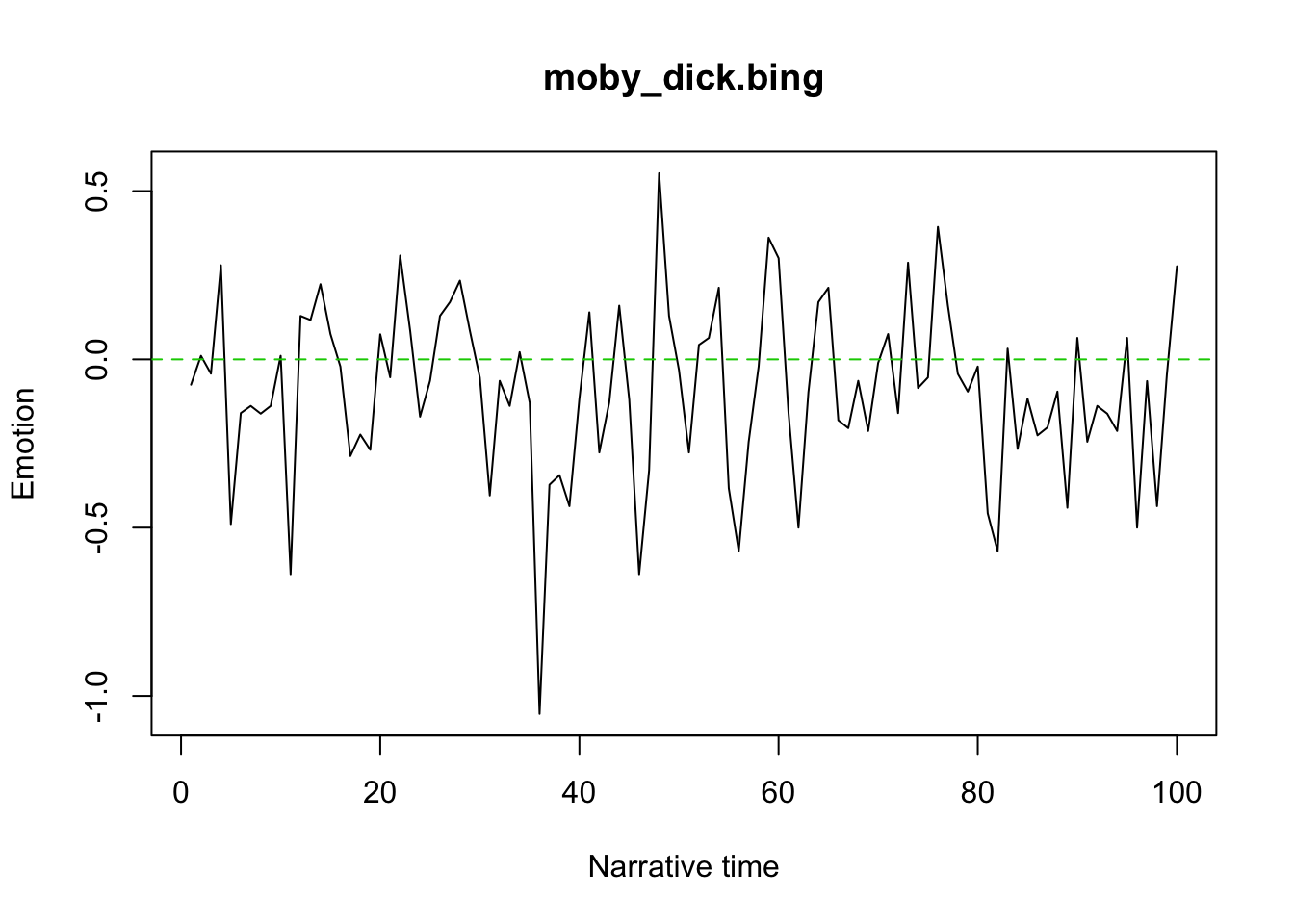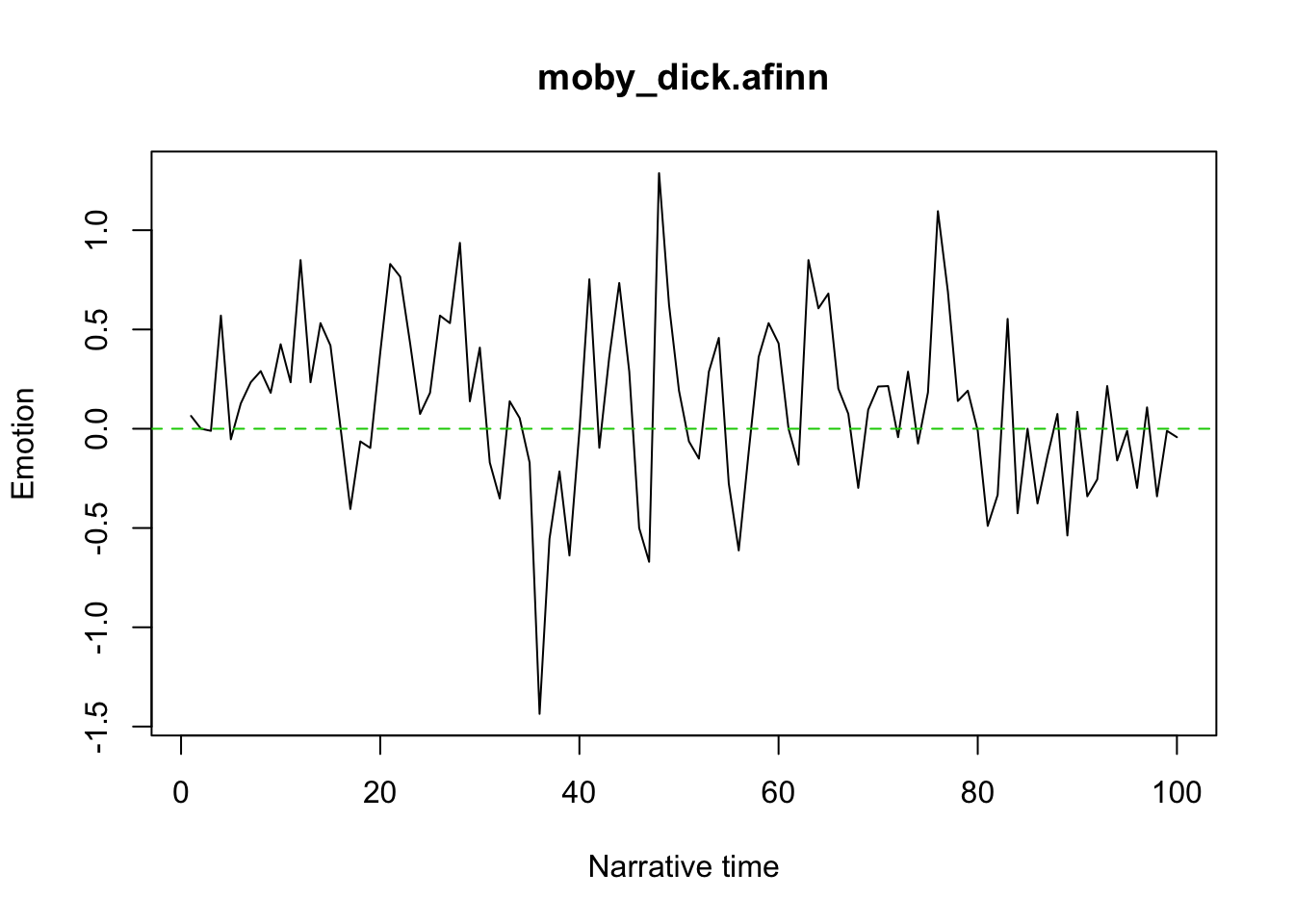Exploring the package syuzhet by Matt Jockers.

``````if(!require("syuzhet")) {
devtools::install_github("mjockers/syuzhet")
library("syuzhet")
}
library("magrittr")
library("rvest")
library("NLP")
library("rlist")
library("tidyr")
library("ggplot2")
library("stringr")``````

Load Damnation of Theron Ware, Moby Dick, Minister’s Wooing, Uncle Tom’s Cabin, and Norwood. Put them in a list and find the sentences. Cache them.

``````if(!file.exists("books.rda")) {
moby_dick <- "mobydick.txt" %>%
get_text_as_string()
theron_ware <- "theronware.txt" %>%
get_text_as_string()
norwood <- "https://archive.org/stream/norwood00beecgoog/norwood00beecgoog_djvu.txt" %>%
html() %>%
html_node("pre") %>%
html_text() %>%
as.String()
wooing <- "wooing.txt" %>%
get_text_as_string()
uncle_tom <- "uncletom.txt" %>%
get_text_as_string()

books <- list(moby_dick = moby_dick,
theron_ware = theron_ware,
norwood = norwood,
wooing = wooing,
uncle_tom = uncle_tom) %>%
lapply(get_sentences)

save(books, file = "books.rda")
} else {
}``````

Run the sentiment analysis using `bing` and `afinn` methods. I couldn’t get `stanford` to work, though I’ve never really tried to get the Stanford NLP to work on this machine before.

``````multi_sentiment <- function(sentences) {
list(bing  = get_sentiment(sentences, method = "bing"),
afinn = get_sentiment(sentences, method = "afinn"),
nrc   = get_sentiment(sentences, method = "nrc")
#        stanford = get_sentiment(sentences, method = "stanford",
#                     path_to_tagger = "/Applications/stanford-corenlp")
)
}``````
``````sentiment <- books %>%
lapply(multi_sentiment)``````

How do these novels compare to one another in summary terms?

``````sum_up_sentiment <- function(x) {
apply_sentiment <- function(vec) {
list(sum = sum(vec),
mean = mean(vec),
summary = summary(vec))
}

if(is.list(x))
lapply(x, apply_sentiment)
else
apply_sentiment(x)
}``````
``````sentiment %>%
lapply(sum_up_sentiment) %>%
list.unzip()``````
``````## \$bing
##         moby_dick   theron_ware norwood   wooing    uncle_tom
## sum     -888        474         2448      2376      120
## mean    -0.09474021 0.07247706  0.2045284 0.5074754 0.01326847
## summary Numeric,6   Numeric,6   Numeric,6 Numeric,6 Numeric,6
##
## \$afinn
##         moby_dick theron_ware norwood   wooing    uncle_tom
## sum     1072      2357        6466      5430      2060
## mean    0.1143711 0.3603976   0.5402289 1.159761  0.2277753
## summary Numeric,6 Numeric,6   Numeric,6 Numeric,6 Numeric,6
##
## \$nrc
##         moby_dick theron_ware norwood   wooing    uncle_tom
## sum     2231      2565        5416      4942      2489
## mean    0.2380241 0.3922018   0.4525023 1.055532  0.2752101
## summary Numeric,6 Numeric,6   Numeric,6 Numeric,6 Numeric,6``````

It’s curious that Moby Dick has a negative mean and sum with `bing` and positive with `afinn`. In general, the `afinn` numbers are much higher than the `bing` numbers. I take this to mean that the numbers generated by the different methods are not meant to be compared. It’s also curious that `afinn` generates all positive numbers and `bing` only has one negative number. I’m not sure how to interpret that right now.

Nevertheless, the ranking by mean is consistent between `bing` and `afinn`: Moby Dick, Uncle Tom’s Cabin, Theron Ware, Norwood, and Wooing. The `nrc` method gives slightly different results. It’s not surprising that Norwood and Wooing are on average more positive than the others.

Now let’s plot sentiment:

``````plot_sentiment <- function(x, title) {
plot(x,
type = "l",
main = title,
xlab = "Narrative time",
ylab = "Emotion",
# ylim = c(-1.5, 3.25) # roughly the min and the max
)
abline(h = 0, col = 3, lty = 2) # neutral sentiment
}``````
``````sentiment %>%
list.flatten() %>%
lapply(get_percentage_values) %>%
Map(plot_sentiment, ., names(.))``````